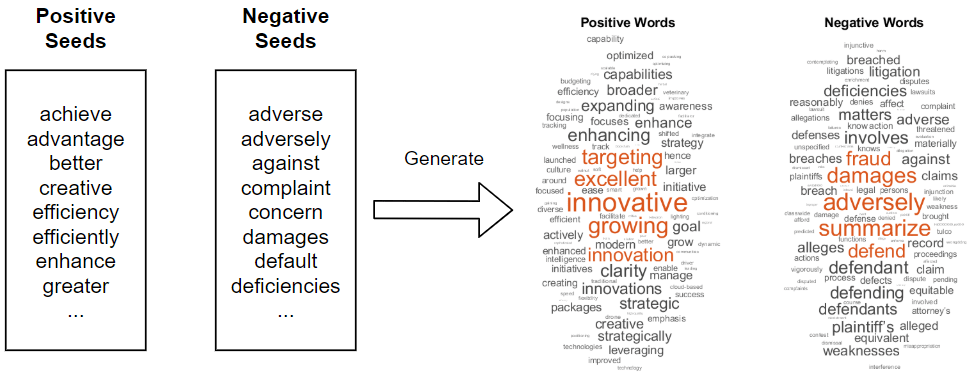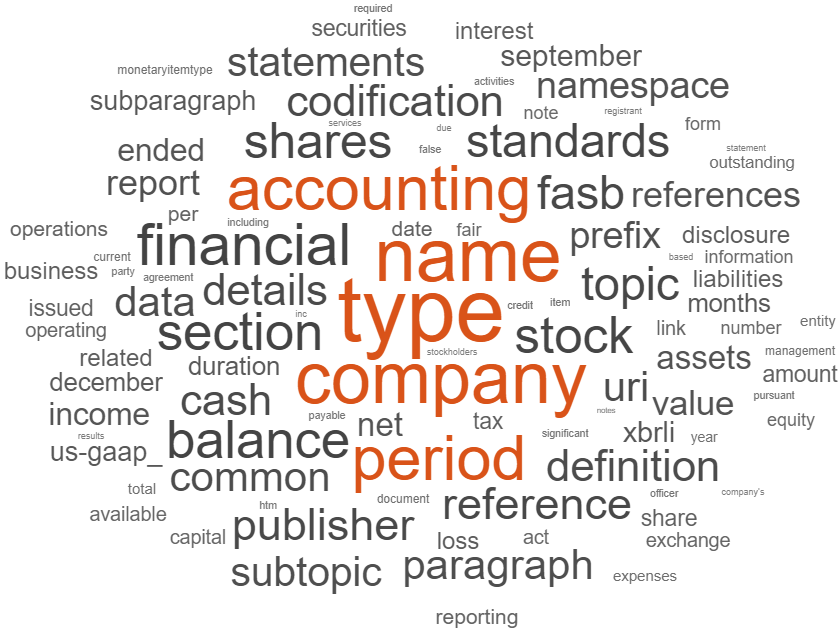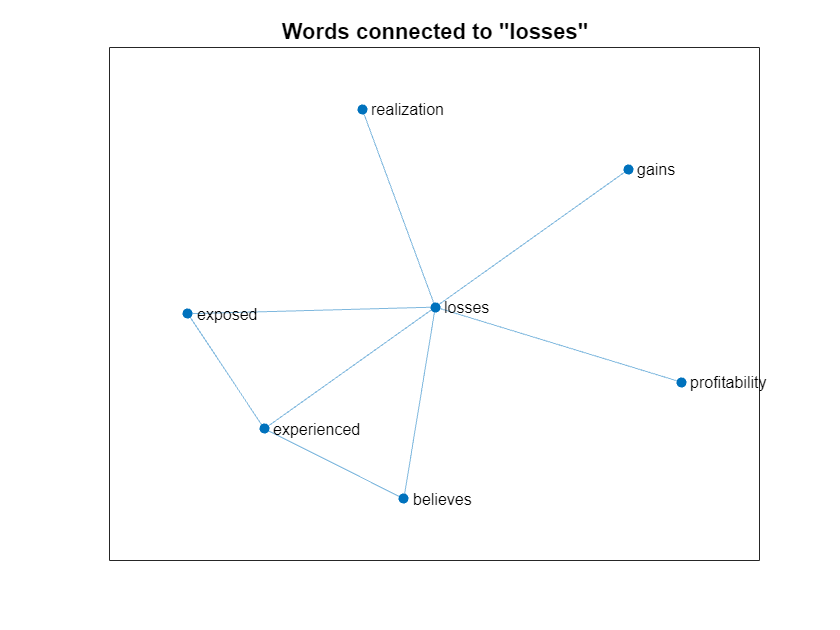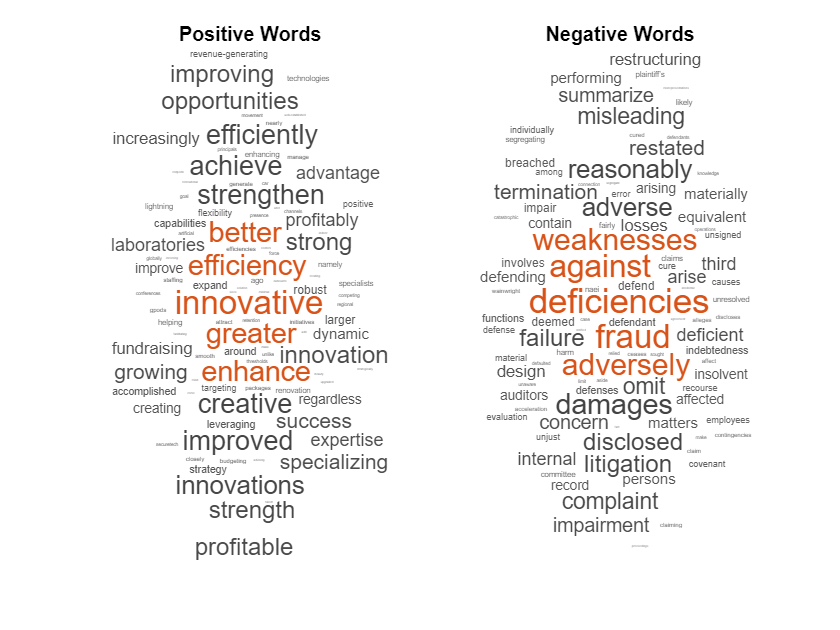# Generate Domain Specific Sentiment Lexicon

This example shows how to generate a lexicon for sentiment analysis using 10-K and 10-Q financial reports.

Sentiment analysis allows you to automatically summarize the sentiment in a given piece of text. For example, assign the pieces of text "This company is showing strong growth." and "This other company is accused of misleading consumers." with positive and negative sentiment, respectively. Also, for example, to assign the text "This company is showing extremely strong growth." a stronger sentiment score than the text "This company is showing strong growth."

Sentiment analysis algorithms such as VADER rely on annotated lists of words called sentiment lexicons. For example, VADER uses a sentiment lexicon with words annotated with a sentiment score ranging from -1 to 1, where scores close to 1 indicate strong positive sentiment, scores close to -1 indicate strong negative sentiment, and scores close to zero indicate neutral sentiment.

To analyze the sentiment of text using the VADER algorithm, use the `vaderSentimentScores` function. If the sentiment lexicon used by the `vaderSentimentScores` function does not suit the data you are analyzing, for example, if you have a domain-specific data set like medical or engineering data, then you can generate your own custom sentiment lexicon using a small set of seed words.

This example shows how to generate a sentiment lexicon given a collection of seed words using a graph-based approach based on :

• Train a word embedding that models the similarity between words using the training data.

• Create a simplified graph representing the embedding with nodes corresponding to words and edges weighted by similarity.

• To determine words with strong polarity, identify the words connected to multiple seed words through short but heavily weighted paths.Download the 10-K and 10-Q financial reports data from Securities and Exchange Commission (SEC) via the Electronic Data Gathering, Analysis, and Retrieval (EDGAR) API  using the `financeReports` helper function attached to this example as a supporting file. To access this file, open this example as a Live Script. The `financeReports` function downloads 10-K and 10-Q reports for the specified year, quarter, and maximum character length.

Download a set of reports from the fourth quarter 2019 with fewer than 2 million characters. Depending on the sizes of the reports, this can take some time to run. If you have Parallel Computing Toolbox™, then the function processes the reports in parallel.

```year = 2019; qtr = 4; maxLength = 2e6; textData = financeReports(year,qtr,maxLength);```
```Downloading 10-K and 10-Q reports... Done. Elapsed time is 605.480268 seconds. ```

Define sets of positive and negative seed words to use with this data.

```seedsPositive = ["achieve" "advantage" "better" "creative" "efficiency" ... "efficiently" "enhance" "greater" "improved" "improving" ... "innovation" "innovations" "innovative" "opportunities" "profitable" ... "profitably" "strength" "strengthen" "strong" "success"]'; seedsNegative = ["adverse" "adversely" "against" "complaint" "concern" ... "damages" "default" "deficiencies" "disclosed" "failure" ... "fraud" "impairment" "litigation" "losses" "misleading" ... "omit" "restated" "restructuring" "termination" "weaknesses"]';```

### Prepare Text Data

Create a function names `preprocessText` that prepares the text data for analysis. The `preprocessText` function, listed at the end of the example performs the following steps:

• Erase any URLs.

• Tokenize the text.

• Remove tokens containing digits.

• Convert the text to lower case.

• Remove any words with two or fewer characters.

• Remove any stop words.

Preprocess the text using the `preprocessText` function. Depending on the size of the text data, this can take some time to run.

`documents = preprocessText(textData);`

Visualize the preprocessed text data in a word cloud.

```figure wordcloud(documents);```### Train Word Embedding

Word embeddings map words in a vocabulary to numeric vectors. These embeddings can capture semantic details of the words so that similar words have similar vectors.

Train a word embedding that models the similarity between words using the training data. Specify a context window of size 25 and discard words that appear fewer than 20 times. Depending on the size of the text data, this can take some time to run.

`emb = trainWordEmbedding(documents,'Window',25,'MinCount',20);`
```Computing Vocabulary. Word count in millions: 10. Vocabulary count: 12648. Training: 100% Loss: 0.713101 Remaining time: 0 hours 0 minutes. ```

### Create Word Graph

Create a simplified graph representing the embedding with nodes corresponding to words and edges weighted by similarity.

Create a weighted graph with nodes corresponding to words in the vocabulary, edges denoting whether the words are within a neigborhood of 7 of each other, and weights corresponding to the cosine distance between the corresponding word vectors in the embedding.

For each word in the vocabulary, find the nearest 7 words and their cosine distances.

```numNeighbors = 7; vocabulary = emb.Vocabulary; wordVectors = word2vec(emb,vocabulary); [nearestWords,dist] = vec2word(emb,wordVectors,numNeighbors);```

To create the graph, use the `graph` function and specify pairwise source and target nodes, and specify their edge weights.

Define the source and target nodes.

```sourceNodes = repelem(vocabulary,numNeighbors); targetNodes = reshape(nearestWords,1,[]);```

Calculate the edge weights.

`edgeWeights = reshape(dist,1,[]);`

Create a graph connecting each word with its neigbors with edge weights corresponding to the similarity scores.

`wordGraph = graph(sourceNodes,targetNodes,edgeWeights,vocabulary);`

Remove the repeated edges using the `simplify` function.

`wordGraph = simplify(wordGraph);`

Visualize the section of the word graph connected to the word "damage".

```word = "damage"; idx = findnode(wordGraph,word); nbrs = neighbors(wordGraph,idx); wordSubgraph = subgraph(wordGraph,[idx; nbrs]); figure plot(wordSubgraph) title("Words connected to """ + word + """")```### Generate Sentiment Scores

To determine words with strong polarity, identify the words connected to multiple seed words through short but heavily weighted paths.

Initialize an array of sentiment scores corresponding to each word in the vocabulary.

`sentimentScores = zeros([1 numel(vocabulary)]);`

Iteratively traverse the graph and update the sentiment scores.

Traverse the graph at different depths. For each depth, calculate the positive and negative polarity of the words by using the positive and negative seeds to propagate sentiment to the rest of the graph.

For each depth:

• Calculate the positive and negative polarity scores.

• Account for the difference in overall mass of positive and negative flow in the graph.

• For each node-word, normalize the difference of its two scores.

After running the algorithm, if a phrase has a higher positive than negative polarity score, then its final polarity will be positive, and negative otherwise.

Specify a maximum path length of 4.

`maxPathLength = 4;`

Iteratively traverse the graph and calculate the sum of the sentiment scores.

```for depth = 1:maxPathLength % Calculate polarity scores. polarityPositive = polarityScores(seedsPositive,vocabulary,wordGraph,depth); polarityNegative = polarityScores(seedsNegative,vocabulary,wordGraph,depth); % Account for difference in overall mass of positive and negative flow % in the graph. b = sum(polarityPositive) / sum(polarityNegative); % Calculate new sentiment scores. sentimentScoresNew = polarityPositive - b * polarityNegative; sentimentScoresNew = normalize(sentimentScoresNew,'range',[-1,1]); % Add scores to sum. sentimentScores = sentimentScores + sentimentScoresNew; end```

Normalize the sentiment scores by the number of iterations.

`sentimentScores = sentimentScores / maxPathLength;`

Create a table containing the vocabulary and the corresponding sentiment scores.

```tbl = table; tbl.Token = vocabulary'; tbl.SentimentScore = sentimentScores';```

To remove tokens with neutral sentiment from the lexicon, remove the tokens with sentiment score that have absolute value less than a threshold of 0.1.

```thr = 0.1; idx = abs(tbl.SentimentScore) < thr; tbl(idx,:) = [];```

Sort the table rows by descending sentiment score and view the first few rows.

```tbl = sortrows(tbl,'SentimentScore','descend'); head(tbl)```
```ans=8×2 table Token SentimentScore _____________ ______________ "innovative" 1 "efficiency" 0.91852 "strong" 0.82362 "efficiently" 0.81475 "creative" 0.74264 "enhance" 0.73791 "innovations" 0.72985 "improved" 0.71476 ```

You can use this table as a custom sentiment lexicon for the `vaderSentimentScores` function.

Visualize the sentiment lexicon in word clouds. Display tokens with a positive score in one word cloud and tokens with negative scores in another. Display the words with sizes given by the absolute value their corresponding sentiment score.

```figure subplot(1,2,1); idx = tbl.SentimentScore > 0; tblPositive = tbl(idx,:); wordcloud(tblTopPositive,'Token','SentimentScore') title('Positive Words') subplot(1,2,2); idx = tbl.SentimentScore < 0; tblNegative = tbl(idx,:); tblNegative.SentimentScore = abs(tblNegative.SentimentScore); wordcloud(tblTopNegative,'Token','SentimentScore') title('Negative Words')```Export the table to a CSV file.

```filename = "financeSentimentLexicon.csv"; writetable(tbl,filename)```

### Analyze Sentiment in Text

To analyze the sentiment in for previously unseen text data, preprocess the text using the same preprocessing steps and use the `vaderSentimentScores` function.

Create a string array containing the text data and preprocess it using the `preprocessText` function.

```textDataNew = [ "This company is showing extremely strong growth." "This other company is accused of misleading consumers."]; documentsNew = preprocessText(textDataNew);```

Evaluate the sentiment using the `vaderSentimentScores` function. Specify the sentiment lexicon created in this example using the `'SentimentLexicon'` option.

`compoundScores = vaderSentimentScores(documentsNew,'SentimentLexicon',tbl)`
```compoundScores = 2×1 0.2834 -0.1273 ```

Positive and negative scores indicate positive and negative sentiment, respectively. The magnitude of the value corresponds to the strength of the sentiment.

### Supporting Functions

#### Text Preprocessing Function

The `preprocessText` function performs the following steps:

• Erase any URLs.

• Tokenize the text.

• Remove tokens containing digits.

• Convert the text to lower case.

• Remove any words with two or fewer characters.

• Remove any stop words.

```function documents = preprocessText(textData) % Erase URLS. textData = eraseURLs(textData); % Tokenize. documents = tokenizedDocument(textData); % Remove tokens containing digits. pat = textBoundary + wildcardPattern + digitsPattern + wildcardPattern + textBoundary; documents = replace(documents,pat,""); % Convert to lowercase. documents = lower(documents); % Remove short words. documents = removeShortWords(documents,2); % Remove stop words. documents = removeStopWords(documents); end```

#### Polarity Scores Function

The `polarityScores` function returns a vector of polarity scores given a set of seed words, vocabulary, graph, and a specified depth. The function computes the sum over the maximum weighted path from every seed word to each node in the vocabulary. A high polarity score indicates phrases connected to multiple seed words via both short and strongly weighted paths.

The function performs the following steps:

• Initialize the scores of the seeds with ones and otherwise zeros.

• Loop over the seeds. For each seed, iteratively traverse the graph at different depth levels. For the first iteration, set the search space to the immediate neighbors of the seed.

• For each depth level, loop over the nodes in the search space and identify its neighbors in the graph.

• Loop over its neighbors and update the corresponding scores. The updated score is the maximum value of the current score for the seed and neighbor, and the score for the seed and search node weighted by the corresponding graph edge.

• At the end of the search for the depth level, append the neighbors to the search space. This increases the depth of the search for the next iteration.

The output polarity is the sum of the scores connected to the input seeds.

```function polarity = polarityScores(seeds,vocabulary,wordGraph,depth) % Initialize scores. vocabularySize = numel(vocabulary); scores = zeros(vocabularySize); idx = ismember(vocabulary,seeds); scores(idx,idx) = eye(numel(seeds)); % Loop over seeds. for i = 1:numel(seeds) % Initialize search space. seed = seeds(i); idxSeed = vocabulary == seed; searchSpace = find(idxSeed); % Search at different depths. for d = 1:depth % Loop over nodes in search space. numNodes = numel(searchSpace); for k = 1:numNodes idxNew = searchSpace(k); % Find neighbors and weights. nbrs = neighbors(wordGraph,idxNew); idxWeights = findedge(wordGraph,idxNew,nbrs); weights = wordGraph.Edges.Weight(idxWeights); % Loop over neighbors. for j = 1:numel(nbrs) % Calculate scores. score = scores(idxSeed,nbrs(j)); scoreNew = scores(idxSeed,idxNew); % Update score. scores(idxSeed,nbrs(j)) = max(score,scoreNew*weights(j)); end % Appended nodes to search space for next depth iteration. searchSpace = [searchSpace nbrs']; end end end % Find seeds in vocabulary. [~,idx] = ismember(seeds,vocabulary); % Sum scores connected to seeds. polarity = sum(scores(idx,:)); end```

### Bibliography

1. Velikovich, Lenid. "The Viability of Web-derived Polarity Lexicons." In Proceedings of The Annual Conference of the North American Chapter of the Association for Computational Linguistics, 2010, pp. 777-785. 2010.

2. Accessing EDGAR Data. https://www.sec.gov/edgar/searchedgar/accessing-edgar-data.htm